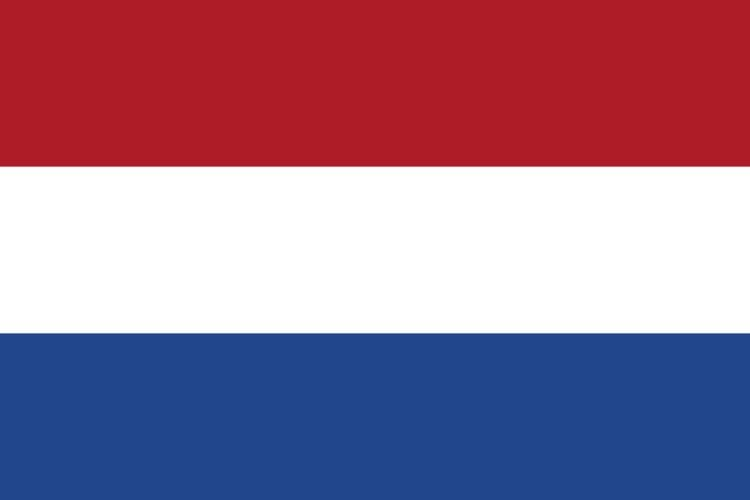# Dutch national flag problem

Updated on
Covid-19The Dutch national flag problem (DNF) is a computer science programming problem proposed by Edsger Dijkstra. The flag of the Netherlands consists of three colors: red, white and blue. Given balls of these three colors arranged randomly in a line (the actual number of balls does not matter), the task is to arrange them such that all balls of the same color are together and their collective color groups are in the correct order.

## Contents

The solution to this problem is of interest for designing sorting algorithms; in particular, variants of the quicksort algorithm that must be robust to repeated elements need a three-way partitioning function that groups items less than a given key (red), equal to the key (white) and greater than the key (blue). Several solutions exist that have varying performance characteristics, tailored to sorting arrays with either small or large numbers of repeated elements.

## The array case

This problem can also be viewed in terms of rearranging elements of an array. Suppose each of the possible elements could be classified into exactly one of three categories (bottom, middle, and top). For example, if all elements are in 0 ... 1, the bottom could be defined as elements in 0 ... 0.1 (not including 0.1), the middle as 0.1 ... 0.3 (not including 0.3) and the top as 0.3 and greater. (The choice of these values illustrates that the categories need not be equal ranges). The problem is then to produce an array such that all "bottom" elements come before (have an index less than the index of) all "middle" elements, which come before all "top" elements.

One algorithm is to have the top group grow down from the top of the array, the bottom group grow up from the bottom, and keep the middle group just above the bottom. The algorithm indexes three locations, the bottom of the top group, the top of the bottom group, and the top of the middle group. Elements that are yet to be sorted fall between the middle and the top group. At each step, examine the element just above the middle. If it belongs to the top group, swap it with the element just below the top. If it belongs in the bottom, swap it with the element just above the bottom. If it is in the middle, leave it. Update the appropriate index. Complexity is Θ(n) moves and examinations.

## Pseudocode

The following pseudocode for three-way partitioning assumes zero-based array indexing. It uses three indices i, j and n, maintaining the invariant that ij. n holds the boundary of numbers greater than mid. j is the position of number under consideration. And i is the boundary for the numbers lesser than the mid.

procedure three-way-partition(A : array of values, mid : value): i ← 0 j ← 0 n ← size of A - 1 while j ≤ n: if A[j] < mid: swap A[i] and A[j] i ← i + 1 j ← j + 1 else if A[j] > mid: swap A[j] and A[n] n ← n - 1 else: j ← j + 1

Note that j will be greater than i only if the mid is hit.

Similar Topics
North 24 Kaatham
Spencer Bohren
Georgi Mamalev
Topics